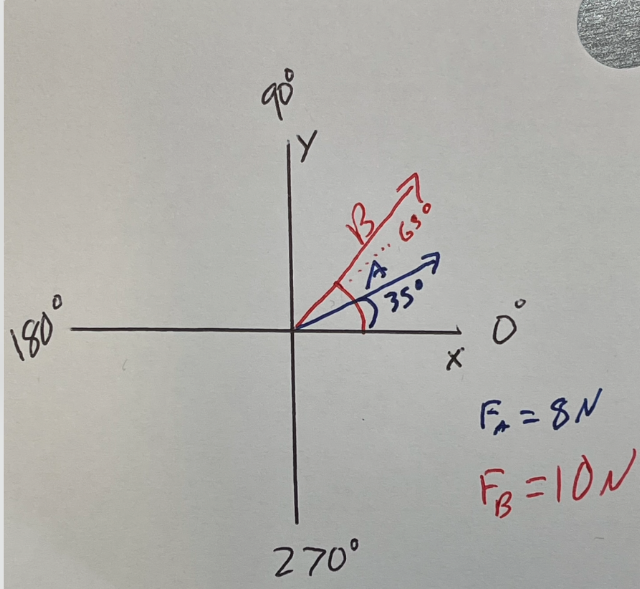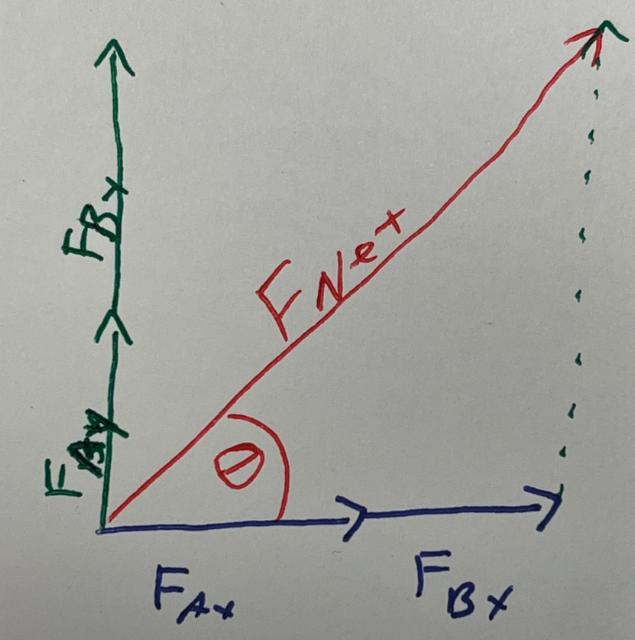## Tuesday, October 19, 2021

Physics Index

(You're not trying to get pushy, are you?)

In physics, a force is any interaction that, when unopposed, will change the motion of an object. In other words, a force can cause an object with mass to change its velocity, i.e., to accelerate. In most situations, there are numerous forces acting on an object at any given time.

Forces are vector quantities. As such, the have magnitudes and directions.

(By the way, forces are measured in Newtons. Newtons are abbreviated as N.)

When you add up forces, you get a resultant, net force. If we agree that F is the abbreviation for forces, then…

Fnet is the net force.

We can denote other forces as A, B, C or 1, 2, 3 or something…

The net force is the vector sum of all the forces.

Since forces have both magnitude and direction, adding them requires dealing with the directions.

### Adding Force Vectors in One Dimensions

In one dimension, adding force vectors is trivial. Decide that one direction in the dimension is positive; that makes the opposite direction negative.

Let's go with left and right…

Force A is 2 N to the right
Force B is 4 N to the left
Force C is 6 N to the right

Decide which is positive and which is negative: Hmmm… let's say left is negative and right is positive

Add them up. If you get a negative for your answer the direction is left and if you get a positive for your answer, then the direction is right.

What would that actually look like…

Fnet = FA + FB + FC
Fnet = 2N + (-4N) + 6N
Fnet = 8N - 4N
Fnet = 4N

Many, many forces can be acting on an object at the same time. If they are all in the same 2 dimensional space, it is just assigning each direction a sign (positive or negative), then adding them up.

Here's an example problem you can do:

A boat is traveling up a river. The motor pushes the boat upstream with a force of 200 N. The current pushes the boat downstream with a force of 10 N. The air resistance of the moving boat creates a force of 5 N in the direction opposite the boat's movement (downstream).

What is the net force on the boat?

### Adding Force Vectors in Two Dimensions

In the real world… Well, aligning all of the forces into two dimensions is uncommon. More likely, forces will be acting on an object at different angles.

The trivial case is when all of the forces are aligned on two perpendicular axes. Let's go with Up-Down and Left-RightFancy illustration 1

In the image, A and B are perpendicular to C and D. The first thing to do is to combine the Up-Down vectors into a single resultant vector, then combine the Left-Right vectors into a single vector.

NOTE: You could have a zillion Up-Down vectors that would ALL combine into one resulting Up-Down vector. Likewise a zillion Left-Right vectors would also combine into one resulting Left-Right vector.

Now, you have two vectors that are at right angles. You can combine them using "The Triangle Method." Essentially…Fancy illustration 2

The not-as-trivial case is when the forces are in non-perpendicular directions. To even describe this, you need to start with some frame of reference. The cartesian coordinate system is a good way to start, to get our bearings regarding this.Fancy illustration 3

Okay, so, now we can get something like this…

Force A has a magnitude of 8 N and acts in a direction that is 35° counterclockwise from the positive x axis.

Sheesh… This does not seem like it will be fun!

It should be noted that the frame of reference can be anything. The cartesian coordinate system is just one that many of us are familiar with.

Going on with the discussion, let's continue…

Force A has a magnitude of 8 N and acts in a direction that is 35° counterclockwise from the positive x axis.

Force B has a magnitude of 10 N and acts in a direction that is 65° counterclockwise from the positive x axis.

So, that would look like this:Fancy illustration 4LINK
So… what is the net force, the resultant force vector? We could use the imprecise graphical
method… But, nah…

What we want to do is break apart the non-perpendicular vectors into perpendicular vectors.

If we had perpendicular component vectors, we could add them up and combine them into two new vectors.

Then, we could use the method examined above to combine the perpendicular vectors.

Let's say it like this (within the cartesian coordinate system):

Step 1: Break down all vectors into x components and y components. (This will require those trig functions won't it?) This will require using trig functions and will be demonstrated in the example that follows. (Sigh… Knew it!)

Step 2: Add all of the x components into a single resultant x vector.

Step 3: Add all of the y components into a single resultant y vector.

Step 4: Now you have two perpendicular components. Do that thing from Fancy Illustration 2 above.

Step 4a: Use the Pythagorean theorem to find the resultant magnitude.
Step 4b: Use inverse tangent to find the angle.

Okay, we should actually do that with the provided example (See Fancy illustration 4.)

Force A has a magnitude of 8 N and acts in a direction that is 35° counterclockwise from the positive x axis.

Force B has a magnitude of 10 N and acts in a direction that is 65° counterclockwise from the positive x axis.

Find the net force, Fnet

STEP 1

We need first to agree that…

FAx is the part of Force A in the x direction. FAy is the component of Force A in the y direction.

That means that, where the magnitude of A is 8 N and the angle is 35,

Sin ø = FAy / FA
Sin 35 = FAy / 8 N
8 N • Sin 35 = FAy
4.6 N = FAy

Cos ø = FAx / FA
Cos 35 = FAx / 8 N
8 • Cos 35 = FAx
6.6 N = FAx

FBx is the part of Force B in the x direction. FBy is the component of Force B in the y direction.

And that means that, where the magnitude of B is 10 N and the angle is 65,

Sin ø = FBy / FB
Sin 65 = FBy / 10 N
10 N • Sin 65 = FBy
9.1 N = FBy

Cos ø = FBx / FB
Cos 65 = FBx / 10 N
10 • Cos 65 = FBx
4.2 N = FBx

STEPS 2 and 3

At this point, we have four vectors: 2 in the x direction and 2 in the y direction. We can add them…

We need another fancy illustration!Fancy illustration 5
Fx = FAx + FBx
F= 6.6 N  + 4.2 N
F= 10.8 N

F= FAy + FBy
F= 4.6 N  + 9.1 N
F= 13.7 N

STEP 4

Refer to Fancy illustration 5.

Step 4a: Use the Pythagorean theorem to find the resultant magnitude.

Fnet2 = Fx2 + Fy2

Fnet2 = 10.8 N 2 + 13.7 N 2

Fnet = 17.4 N

Step 4b:
Use inverse tangent to find the angle.

Tan øFy / Fx
ø = Tan-1 (Fy / Fx)
ø = Tan-1 (13.7 N / 10.8 N)
ø = 51.8°

Ta da! The resulting net force is 17.4 N at an angle of 51.8° counterclockwise to the x axis.